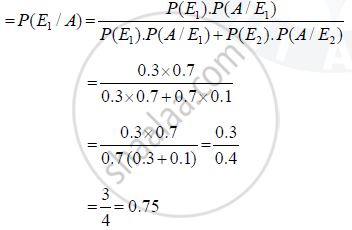Share

# Of the Students in a School, It is Known that 30% Have 100% Attendance and 70% Students Are Irregular at the End of the Year, One Student is Chos~N at Random from the School and He Was Found ·To Have an a Grade. What is the Probability that the Student Has 100% Attendance? is Regularity Required Only in School? Justify Your Answer - CBSE (Science) Class 12 - Mathematics

#### Question

Of the students in a school, it is known that 30% have 100% attendance and 70% students are irregular. Previous year results report that 70% of all students who have 100% attendance attain A grade and 10% irregular students attain A grade in their annual examination. At the end of the year, one student is chos~n at random from the school and he was found ·to have an A grade. What is the probability that the student has 100% attendance? Is regularity required only in school? Justify your answer

#### Solution

Let E_1 is event of students which have 100% attendance and E_2 is event of students which are Irregular

then P(E_1) = 0.3

P(E_2) = 0.7

Let A : Event of students which attendance A grade

then P(A/ E1) = 0.7 and P(A/ E2) = 0.1

So By Bays theorem

P(Probability that student has 100% Attendance)As per answer, the probability of regular students is more than 50%. So the regularty is required.

Is there an error in this question or solution?

#### APPEARS IN

Solution Of the Students in a School, It is Known that 30% Have 100% Attendance and 70% Students Are Irregular at the End of the Year, One Student is Chos~N at Random from the School and He Was Found ·To Have an a Grade. What is the Probability that the Student Has 100% Attendance? is Regularity Required Only in School? Justify Your Answer Concept: Baye'S Theorem.
S